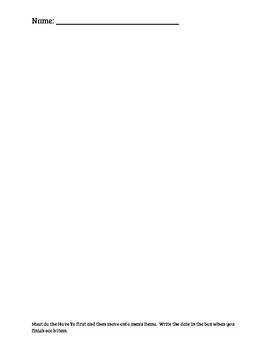Subject
Resource Type
File Type

PDF

(655 KB)
Product Rating
Standards
• Product Description
• StandardsNEW

Math Menus are a way to engage your learners in practice of previously taught math skills allowing you to meet with small groups and individuals highlighting a guided math model. Learners are not required to finish the menu in a week's time, rather over time. You decide if there should be a daily 'have-to' and what that consists of. More importantly, they are held accountable for their daily choices.

Description of activities:

• Daily 'Have-to': Activity aligned to your whole group lesson.
• C-Rods: Students free explore Cuisenaire rods.
• Computer Lesson/Games: Students practice important skills using technology.
• Pattern Blocks Challenge: How many different shapes can you build with 9 blocks?
• Number Writing Practice: Students are provided with a variety of differentiated activities to practice their symbolic notation (chalk, shaving cream, sheets, sand, etc.)
• Math Folders: Students individualized work and/or unfinished work are found in these folders.
• Make 10: Using a deck of cards with the face cards (except for the Joker) removed, students place the the cards face down in rows. Much like Concentration, the goal is to flip over two cards whose sum equals ten. Cards can be placed face up as an accommodation.
• Number Stories: Learners write addition or subtraction number stories in their math journals, including a math drawing and number sentence.
• Weather Graph: Students use the classroom monthly weather graph to answer the weekly question. I ask the students to answer in their math journals but you could certainly use multiple ways to show their understanding. My questions include: Which type of weather has the most days? Which has the fewest? How many more days are cloudy than sunny?
• Student Game: This was student generated. A learner created a game and asked if they could teach their peers how to play. They simply created a number path and the object was to roll the die and move that many spaces, then correctly say the number. Other learners have created games since and we have made it a menu item.
Model with mathematics. Mathematically proficient students can apply the mathematics they know to solve problems arising in everyday life, society, and the workplace. In early grades, this might be as simple as writing an addition equation to describe a situation. In middle grades, a student might apply proportional reasoning to plan a school event or analyze a problem in the community. By high school, a student might use geometry to solve a design problem or use a function to describe how one quantity of interest depends on another. Mathematically proficient students who can apply what they know are comfortable making assumptions and approximations to simplify a complicated situation, realizing that these may need revision later. They are able to identify important quantities in a practical situation and map their relationships using such tools as diagrams, two-way tables, graphs, flowcharts and formulas. They can analyze those relationships mathematically to draw conclusions. They routinely interpret their mathematical results in the context of the situation and reflect on whether the results make sense, possibly improving the model if it has not served its purpose.
Compose two-dimensional shapes (rectangles, squares, trapezoids, triangles, half-circles, and quarter-circles) or three-dimensional shapes (cubes, right rectangular prisms, right circular cones, and right circular cylinders) to create a composite shape, and compose new shapes from the composite shape.
Add and subtract within 20, demonstrating fluency for addition and subtraction within 10. Use strategies such as counting on; making ten (e.g., 8 + 6 = 8 + 2 + 4 = 10 + 4 = 14); decomposing a number leading to a ten (e.g., 13 - 4 = 13 - 3 - 1 = 10 - 1 = 9); using the relationship between addition and subtraction (e.g., knowing that 8 + 4 = 12, one knows 12 - 8 = 4); and creating equivalent but easier or known sums (e.g., adding 6 + 7 by creating the known equivalent 6 + 6 + 1 = 12 + 1 = 13).
Count to 120, starting at any number less than 120. In this range, read and write numerals and represent a number of objects with a written numeral.
Organize, represent, and interpret data with up to three categories; ask and answer questions about the total number of data points, how many in each category, and how many more or less are in one category than in another.
Total Pages
N/A
N/A
Teaching Duration
N/A
Report this Resource to TpT
Reported resources will be reviewed by our team. Report this resource to let us know if this resource violates TpT’s content guidelines.